Courses

# Two Body Collisions in Center of Mass Frame - Collisions, Classical Mechanics, CSIR-NET Physical Sc Physics Notes | EduRev

## Physics for IIT JAM, UGC - NET, CSIR NET

Created by: Akhilesh Thakur

## Physics : Two Body Collisions in Center of Mass Frame - Collisions, Classical Mechanics, CSIR-NET Physical Sc Physics Notes | EduRev

The document Two Body Collisions in Center of Mass Frame - Collisions, Classical Mechanics, CSIR-NET Physical Sc Physics Notes | EduRev is a part of the Physics Course Physics for IIT JAM, UGC - NET, CSIR NET.
All you need of Physics at this link: Physics

In physics, the center-of-momentum frame (also zero-momentum frame or COM frame) of a system is the unique (up to velocity but not origin) inertial frame in which the total momentum of the system vanishes. The center of momentum of a system is not a location (but a collection of relative momenta/velocities). Thus "center of momentum" means "center-of-momentum frame" and is a short form of this phrase.

A special case of the center-of-momentum frame is the center-of-mass frame: an inertial frame in which the center of mass (which is a physical point) remains at the origin. In all COM frames, the center of mass is at rest, but it is not necessarily at the origin of the coordinate system.

In special relativity, the COM frame is necessarily unique only when the system is isolated.

Properties

General

The center of momentum frame is defined as the inertial frame in which the sum over the linear momentum of each particle vanishes. Let S denote the laboratory reference system and S′ denote the center-of-momentum reference frame. Using a galilean transformation, the particle velocity in S′ is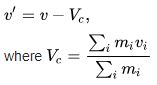is the velocity of the mass center. The total momentum in the center-of-momentum system then vanishes: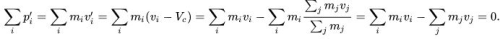Also, the total energy of the system is the minimal energy as seen from all inertial reference frames.

Special relativity

In relativity, COM frame exists for an isolated massive system. This is a consequence of Noether's theorem. In the COM frame the total energy of the system is the rest energy, and this quantity (when divided by the factor c2, where c is the speed of light) gives the rest mass (invariant mass) of the system: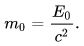The invariant mass of the system is given in any inertial frame by the relativistic invariant relation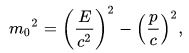but for zero momentum the momentum term (p/c)2 vanishes and thus the total energy coincides with the rest energy.

Systems that have nonzero energy but zero rest mass (such as photons moving in a single direction, or equivalently, plane electromagnetic waves) do not have COM frames, because there is no frame in which they have zero net momentum. Due to the invariance of the speed of light, such massless systems must travel at the speed of light in any frame, and therefore always possess a net momentum magnitude that is equal to their energy divided by the speed of light: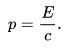Two-body problem

An example of the usage of this frame is given below – in a two-body collision, not necessarily elastic (where kinetic energy is conserved). The COM frame can be used to find the momentum of the particles much easier than in a lab frame: the frame where the measurement or calculation is done. The situation is analyzed using Galilean transformations and conservation of momentum (for generality, rather than kinetic energies alone), for two particles of mass m1 and m2, moving at initial velocities (before collision) u1 and u2 respectively. The transformations are applied to take the velocity of the frame from the velocity of each particle from the lab frame (unprimed quantities) to the COM frame (primed quantities):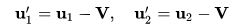where V is the velocity of the COM frame. Since V is the velocity of the COM, i.e. the time derivative of the COM location R (position of the center of mass of the system):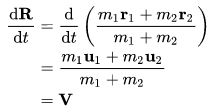so at the origin of the COM frame, R0, this implies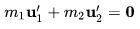The same results can be obtained by applying momentum conservation in the lab frame, where the momenta are p1 and p2: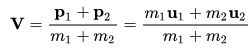and in the COM frame, where it is asserted definitively that the total momenta of the particles, p1' and p2', vanishes: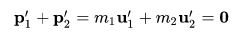Using the COM frame equation to solve for V returns the lab frame equation above, demonstrating any frame (including the COM frame) may be used to calculate the momenta of the particles. It has been established that the velocity of the COM frame can be removed from the calculation using the above frame, so the momenta of the particles in the COM frame can be expressed in terms of the quantities in the lab frame (i.e. the given initial values):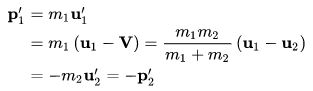notice the relative velocity in the lab frame of particle 1 to 2 is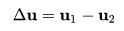and the 2-body reduced mass is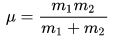so the momenta of the particles compactly reduce to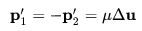This is a substantially simpler calculation of the momenta of both particles; the reduced mass and relative velocity can be calculated from the initial velocities in the lab frame and the masses, and the momentum of one particle is simply the negative of the other. The calculation can be repeated for final velocities v1 and v2 in place of the initial velocities u1 and u2, since after the collision the velocities still satisfy the above equations: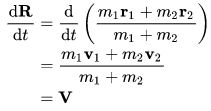so at the origin of the COM frame, R0, this implies after the collision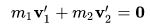In the lab frame, the conservation of momentum fully reads: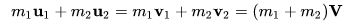This equation does not imply that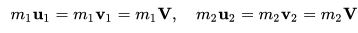instead, it simply indicates the total mass M multiplied by the velocity of the centre of mass V is the total momentum P of the system: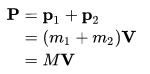Similar analysis to the above obtains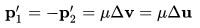where the final relative velocity in the lab frame of particle 1 to 2 is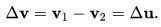159 docs

,

,

,

,

,

,

,

,

,

,

,

,

,

,

,

,

,

,

,

,

,

,

,

,

,

,

,

;